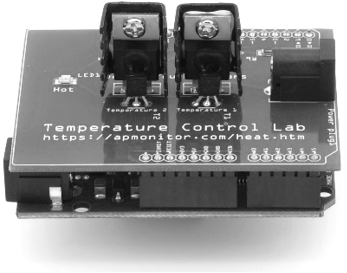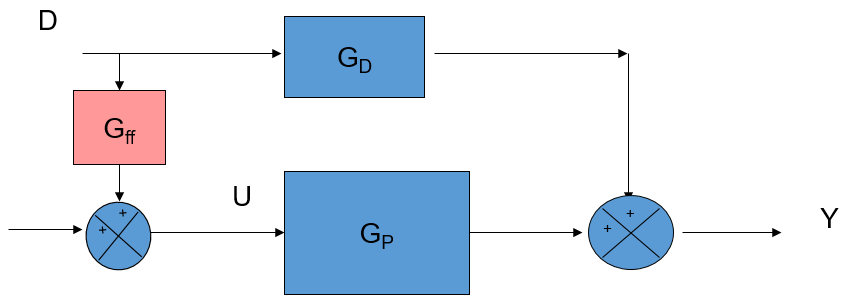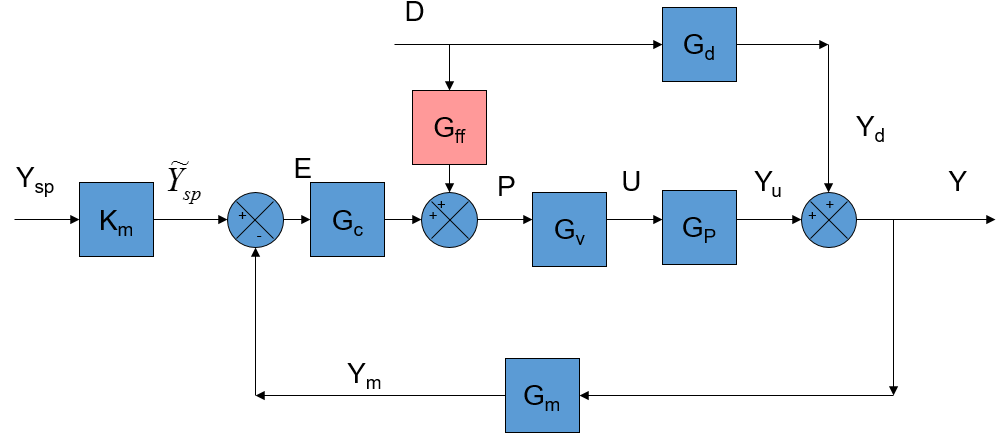## Feedforward ControlFeedforward control is a strategy to reject persistent disturbances that cannot adequately be rejected with feedback control. Feedforward control is typically added to feedback control and is not usually implemented alone. In certain situations, the performance of control systems can be enhanced greatly by the application of feedforward control. Two key characteristics are needed:

1. An identifiable disturbance is affecting significantly the measured variable, in spite of the attempts of a feedback control system to regulate these effects
2. This disturbance can be measured, perhaps with the addition of instrumentation

An ideal feedforward controller is the negative ratio of the disturbance transfer function divided by the process transfer function.

$$G_{ff} = -\frac{G_d}{G_p}$$The process model:

$$\tau_p \frac{dy_1(t)}{dt} = -y_1(t) + K_p u\left(t-\theta_p\right)$$

and the disturbance model:

$$\tau_d \frac{dy_2(t)}{dt} = -y_2(t) + K_d u\left(t-\theta_d\right)$$

can be combined with y(t)=y_1(t)+y_2(t) because they are linear function in deviation variable form. If they have the same time constant \tau_p=\tau_d then the equations can be combined with variables y(t) and u(t) and 5 unknown parameters.

$$K_p \quad \mathrm{= Process \; gain}$$

$$K_d \quad \mathrm{= Disturbance \; gain}$$

$$\tau=\tau_p=\tau_d \quad \mathrm{= Time \; constant}$$

$$\theta_p \quad \mathrm{= Process \; dead \; time}$$

$$\theta_d \quad \mathrm{= Disturbance \; dead \; time}$$

For most implementations, the feedforward control gain is set to a ratio of the disturbance and process gains. This is a good approximation to G_{ff} when the disturbance and controller output act on the process with similar dynamics \tau_d \approx \tau_p. This is often the case in chemical process control applications where the disturbance and the actuator are both physical inputs to the same control volume.

$$K_{ff} = -\frac{K_d}{K_p}$$

Feedforward trim is added to a PID controller with the addition of a final term where d is the measured disturbance.

$$u(t) = u_{bias} + K_c \, e(t) + \frac{K_c}{\tau_I}\int_0^t e(t)dt - K_c \tau_D \frac{d(PV)}{dt} + \color{blue}{K_{ff}\,d}$$

Exercise

A feedforward controller G_{ff} is proposed to improve the control of the disturbance D with the following transfer functions.

$$G_p(s)=\frac{0.6e^{-37s}}{39s+1} \quad \quad G_d(s)=\frac{0.25e^{-57s}}{31s+1} \quad \quad G_v(s)=\frac{5}{2s+1}$$Derive a dynamic G_{ff} and static K_{ff} feedforward controller that will best reject the disturbance.

Assignment